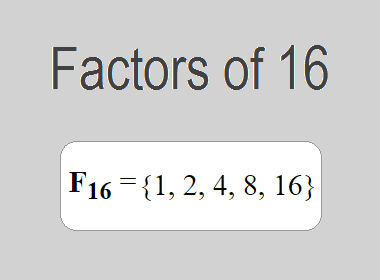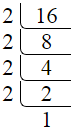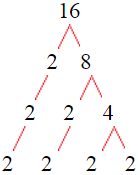# Factors of 16The factors of 16 are 1, 2, 4, 8, and 16 i.e. F16 = {1, 2, 4, 8, 16}. The factors of 16 are all the numbers that can divide 16 without leaving a remainder.

We can check if these numbers are factors of 16 by dividing 16 by each of them. If the result is a whole number, then the number is a factor of 16. Let's do this for each of the numbers listed above:

·        1 is a factor of 16 because 16 divided by 1 is 16.

·        2 is a factor of 16 because 16 divided by 2 is 8.

·        4 is a factor of 16 because 16 divided by 4 is 4.

·        8 is a factor of 16 because 16 divided by 8 is 2.

·        16 is a factor of 16 because 16 divided by 16 is 1.

## How to Find Factors of 16?

1 and the number itself are the factors of every number. So, 1 and 16 are two factors of 16. To find the other factors of 16, we can start by dividing 16 by the numbers between 1 and 16. If we divide 16 by 2, we get a remainder of 0. Therefore, 2 is a factor of 16. If we divide 16 by 3, we get a remainder of 1. Therefore, 3 is not a factor of 16.

Next, we can check if 4 is a factor of 16. If we divide 16 by 4, we get a remainder of 0. Therefore, 4 is also a factor of 16. We can continue this process for all the possible factors of 16.

Through this process, we can find that the factors of 16 are 1, 2, 4, 8, and 16. These are the only numbers that can divide 16 without leaving a remainder.

********************

********************

## Properties of the Factors of 16

The factors of 16 have some interesting properties. One of the properties is that the sum of the factors of 16 is equal to 31. We can see this by adding all the factors of 16 together:

1 + 2 + 4 + 8 + 16 = 31

Another property of the factors of 16 is that the prime factor of 16 is 2 only.

## Applications of the Factors of 16

The factors of 16 have several applications in mathematics. One of the applications is in finding the highest common factor (HCF) of two or more numbers. The HCF is the largest factor that two or more numbers have in common. For example, to find the HCF of 16 and 40, we need to find the factors of both numbers and identify the largest factor they have in common. The factors of 16 are 1, 2, 4, 8, and 16. The factors of 40 are 1, 2, 4, 5, 8, 10, 20, and 40. The largest factor that they have in common is 8. Therefore, the HCF of 16 and 40 is 8.

Another application of the factors of 16 is in prime factorization. Prime factorization is the process of expressing a number as the product of its prime factors. The only prime factor of 16 is 2. Therefore, we can express 16 as:

16 = 2 × 2 × 2 × 2

We can do prime factorization by division and factor tree method also. Here is the prime factorization of 16 by division method,16 = 2 × 2 × 2 × 2

Here is the prime factorization of 16 by the factor tree method,16 = 2 × 2 × 2 × 2

## Conclusion

The factors of 16 are the numbers that can divide 16 without leaving a remainder. The factors of 16 are 1, 2, 4, 8, and 16. The factors of 16 have some interesting properties, such as having a sum of 31. The factors of 16 have several applications in mathematics, such as finding the highest common factor and prime factorization.